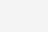``````import exifread
import re

# 读取图片为二进制格式
f = open("luotuo.JPG","rb")

# GPS信息
GPS = {}

# 拍摄时间
Data = ""

for tag,value in tags.items():
# 获取纬度信息
if re.match('GPS GPSLatitude', tag):
try:
match_result=re.match('\[(\w*), (\w*), (\w.*)/(\w.*)\]', str(value)).groups()
GPS['纬度'] = str(int(match_result)) + " " + str(int(match_result)) + " " + str(int(match_result)/int(match_result))
except:
GPS['纬度'] = str(value)
# 获取纬度信息
elif re.match('GPS GPSLongitude', tag):
try:
match_result=re.match('\[(\w*), (\w*), (\w.*)/(\w.*)\]',str(value)).groups()
GPS['经度'] = str(int(match_result)) + " " + str(int(match_result)) + " " + str(int(match_result)/int(match_result))
except:
GPS['经度'] = str(value)
# 获取高度
elif re.match('GPS GPSAltitude', tag):
GPS['高度'] = str(value)
# 获取拍摄时间
elif re.match('Image DateTime', tag):
Data = str(value)

# 打印信息
print("纬 经 度：" + GPS['纬度'] + "," + GPS['经度'])
print("拍摄时间：" + Data)
``````